Test: Multiple Integrals

# Test: Multiple Integrals

Test Description

## 15 Questions MCQ Test Engineering Mathematics | Test: Multiple Integrals

Test: Multiple Integrals for Civil Engineering (CE) 2023 is part of Engineering Mathematics preparation. The Test: Multiple Integrals questions and answers have been prepared according to the Civil Engineering (CE) exam syllabus.The Test: Multiple Integrals MCQs are made for Civil Engineering (CE) 2023 Exam. Find important definitions, questions, notes, meanings, examples, exercises, MCQs and online tests for Test: Multiple Integrals below.
Solutions of Test: Multiple Integrals questions in English are available as part of our Engineering Mathematics for Civil Engineering (CE) & Test: Multiple Integrals solutions in Hindi for Engineering Mathematics course. Download more important topics, notes, lectures and mock test series for Civil Engineering (CE) Exam by signing up for free. Attempt Test: Multiple Integrals | 15 questions in 45 minutes | Mock test for Civil Engineering (CE) preparation | Free important questions MCQ to study Engineering Mathematics for Civil Engineering (CE) Exam | Download free PDF with solutions
 1 Crore+ students have signed up on EduRev. Have you?
Test: Multiple Integrals - Question 1

### The following surface integral is to be evaluated over a sphere for the given steady vector field, F = xi + yj + zk defined with respect to a Cartesian coordinate system having i, j, and k as unit base vectors.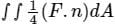, Where S is the sphere, x2 + y2 + z2 = 1 and n is the outward unit normal vector to the sphere. The value of the surface integral is

Detailed Solution for Test: Multiple Integrals - Question 1

Concept:

Gauss divergence theorem:

It states that the surface integral of the normal component of a vector function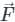taken over a closed surface ‘S’ is equal to the volume integral of the divergence of that vector functiontaken over a volume enclosed by the closed surface ‘S’.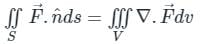Calculation:

Given:

F = xi + yj + zk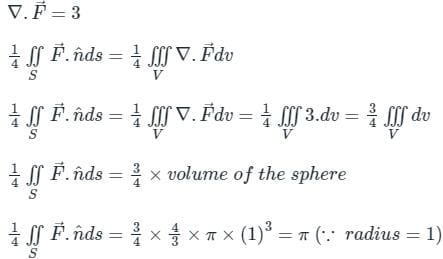Stokes theorem:

It states that the line integral of a vector fieldaround any closed surface C is equal to the surface integral of the normal component of the curl of vectorover an unclosed surface ‘S’.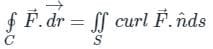Green's theorem: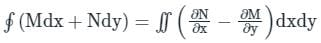*Answer can only contain numeric values
Test: Multiple Integrals - Question 2

### The value of the integral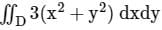, where D is the shaded triangular region shown in the diagram, is _____ (rounded off to the nearest integer).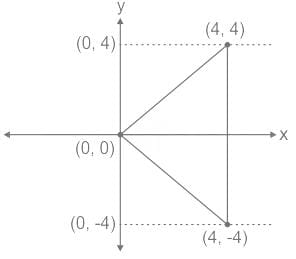Detailed Solution for Test: Multiple Integrals - Question 2

Calculation:

x → 0 to 4

y → -x to x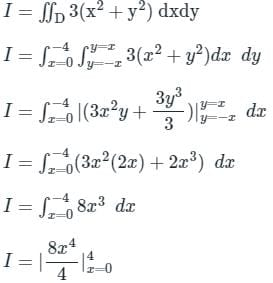I = 2× 44 = 512

*Answer can only contain numeric values
Test: Multiple Integrals - Question 3

### A definite double integral is given below, then, evaluation of the double integral over the region R will be __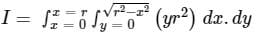Where R is the region on X - Y plane for the function given as,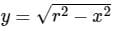and r ∈ [0, 5]

Detailed Solution for Test: Multiple Integrals - Question 3

Concept:

Let’s change the Cartesian coordinate limits into cylindrical planar polar coordinate,

⇒ x = r cosθ; y = r sinθ

Replace dx.dy = rdθ .dr

Calculation:

Given: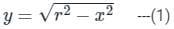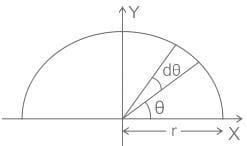θ → 0 to π; r → 0 to 5

Then the limit of the double integration will be,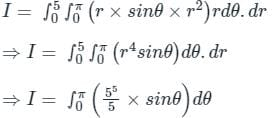⇒ I = - [cos π – cos 0] × 625

I = 1250

Test: Multiple Integrals - Question 4

Evaluate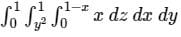Detailed Solution for Test: Multiple Integrals - Question 4

Concept:

The triple integral can be evaluated as repeated integral: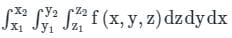First, f(x, y, z) is integrated w.r.t z between limits z1 and z2 keeping x and y constant, then integrated w.r.t y between limits y1 and y2 keeping x constant.
The result is then integrated w.r.t x.

Calculation:

Given: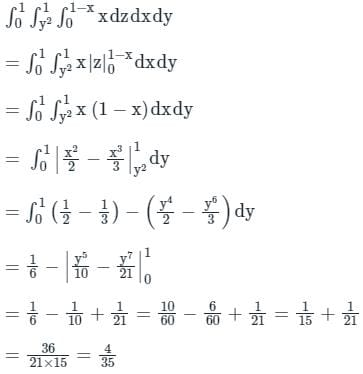Test: Multiple Integrals - Question 5

The value of integral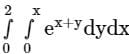is

Detailed Solution for Test: Multiple Integrals - Question 5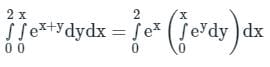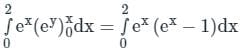Test: Multiple Integrals - Question 6

Evaluate the following integral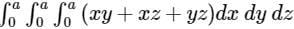.

Detailed Solution for Test: Multiple Integrals - Question 6

Concept:

Triple integral can be evaluated as repeated integral: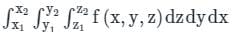First, f(x, y, z) is integrated w.r.t z between limits z1 and z2 keeping x and y constant, then integrated w.r.t y between limits y1 and y2 keeping x constant. The result is then integrated w.r.t x.

Calculation: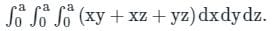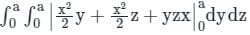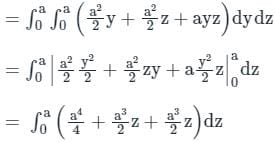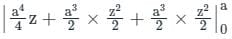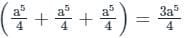*Answer can only contain numeric values
Test: Multiple Integrals - Question 7

The integral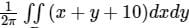, where D denotes the disc ��2 + ��2 ≤ 4, evaluates to__________.

Detailed Solution for Test: Multiple Integrals - Question 7

Given,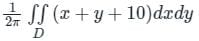and x2 + y2 ≤ 4

Putting x = r.cosθ, y = r.sinθ and dx.dy = r.dr.dθ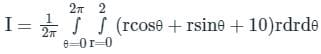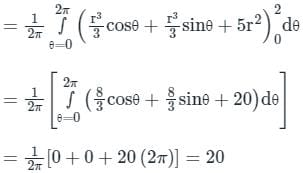Test: Multiple Integrals - Question 8

The area bounded by the curves y2 = 9x, x – y + 2 = 0 is given by

Detailed Solution for Test: Multiple Integrals - Question 8

Calculation

Given equations are: y2 = 9x, x – y + 2 = 0

By solving the above two equations,

The point of intersection of the two curves are: (1, 3) and (4, 6)

Now, the graph is shown below.

By considering the horizontal strip,

The limits of y are:  3 to 6

The limits of x are: (y – 2) to y2/9

Now, the required area is =∫∫dxdy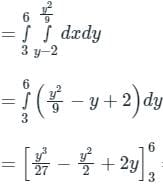= 1/2

Test: Multiple Integrals - Question 9

The area of an ellipse represented by an equation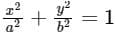is

Detailed Solution for Test: Multiple Integrals - Question 9

Concept:

Ellipse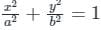Length major axis of ellipse = 2a

Length of minor axis of ellipse = 2b

Area =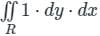Calculation: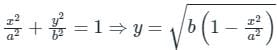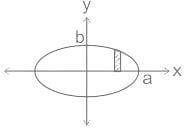For the first quadrant, take a vertical strip as shown. Here, y coordinate varies from 0 to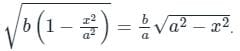Also, the x-coordinate varies from 0 to a

∴ Area =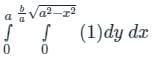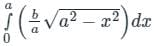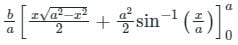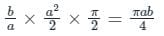∴ The total area of ellipse = 4 × πab/4 = πab units

Test: Multiple Integrals - Question 10

The integral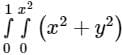equals to

Detailed Solution for Test: Multiple Integrals - Question 10

We have the integration given as,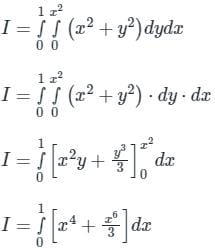Placing the limits we get,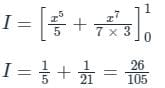Hence the required value of integration will be 26/105.

Test: Multiple Integrals - Question 11

Change the order of integration in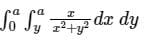Detailed Solution for Test: Multiple Integrals - Question 11

Concept:

In a double integral with variable limits, the change of order of integration can sometimes make the evaluation easy.

Calculation:

Since the limit of y is constant hence, we can say that a horizontal strip was taken for integration now for change of order we will take vertical strip as shown in the following diagram.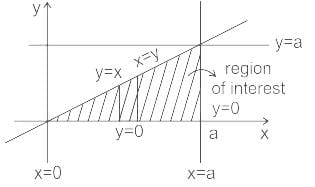∴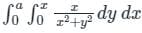is the correct solution.

Test: Multiple Integrals - Question 12

The area between the parabolas y2 = 4ax and x2 = 4ay is

Detailed Solution for Test: Multiple Integrals - Question 12

y2 = 4ax & x2 = 4ay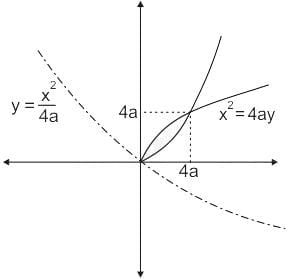We have to find shaded region area.

So area drawn by y= x2 / 4a on x-axis = A1 (say)

Then shaded area = |A1 – A2|

So,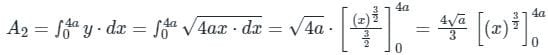⇒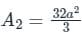⇒ Shaded area =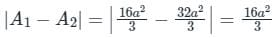*Answer can only contain numeric values
Test: Multiple Integrals - Question 13

Let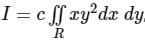, where R is the region shown in the figure and c = 6 × 10-4. The value of I equals________. (Give the answer up to two decimal places.)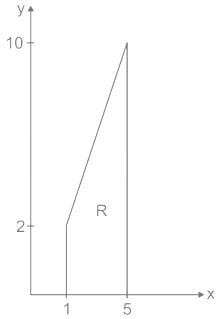Detailed Solution for Test: Multiple Integrals - Question 13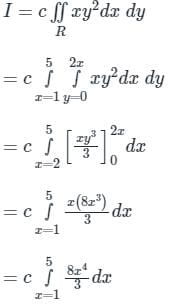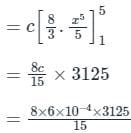= 1

*Answer can only contain numeric values
Test: Multiple Integrals - Question 14

An ellipse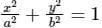is revolved around the y-axis. The volume generated by the solid of revolution if a = 3 and b = 2 is______

Detailed Solution for Test: Multiple Integrals - Question 14

Concept:

The volume of solid generated by revolving around y-axis

V = ∫πx2dy         ----(1)

Volume generated by revolving around x-axis

V = ∫πy2dx         ----(2)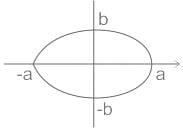Calculations:

From the equation of ellipse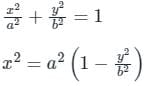Subtitling in (1)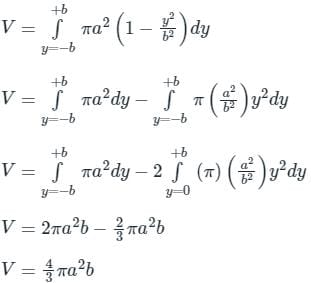Given

a = 3

b = 2

V = 43π322 = 24π

= 75.39

Test: Multiple Integrals - Question 15

The value of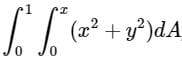, where dA indicate small area in xy - plane, is

Detailed Solution for Test: Multiple Integrals - Question 15

Concept: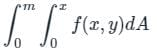Since the inside limit is in terms of x, therefore we have to integrate first the 'y' terms and convert whole expression in terms of x.

Calculation:

Given: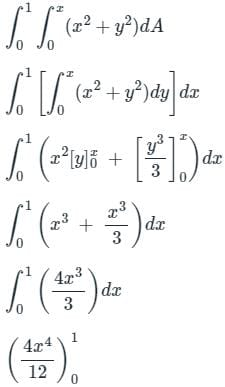= 1/3

## Engineering Mathematics

47 videos|119 docs|75 tests
 Use Code STAYHOME200 and get INR 200 additional OFF Use Coupon Code
Information about Test: Multiple Integrals Page
In this test you can find the Exam questions for Test: Multiple Integrals solved & explained in the simplest way possible. Besides giving Questions and answers for Test: Multiple Integrals, EduRev gives you an ample number of Online tests for practice

## Engineering Mathematics

47 videos|119 docs|75 tests